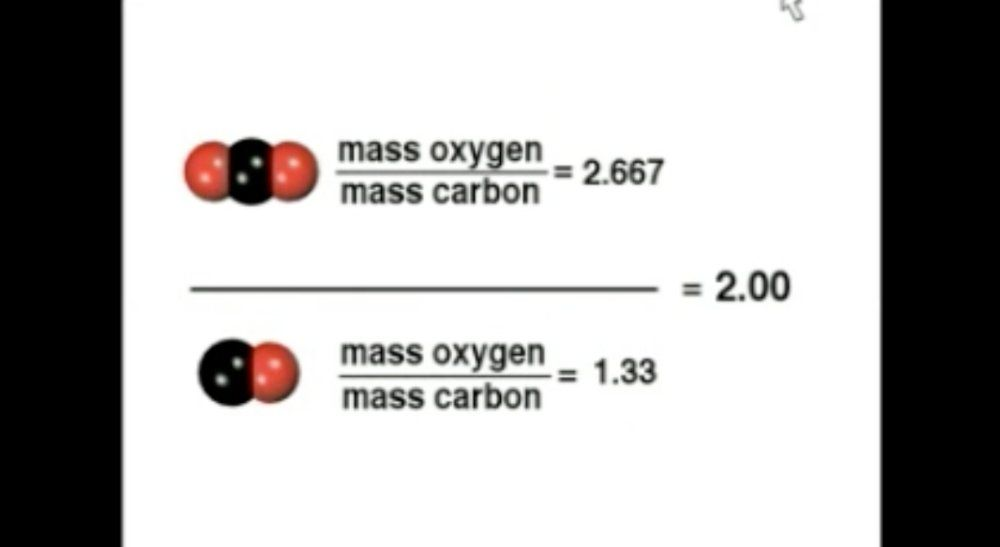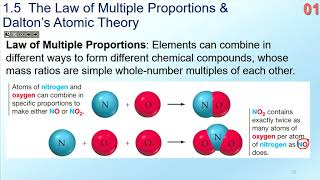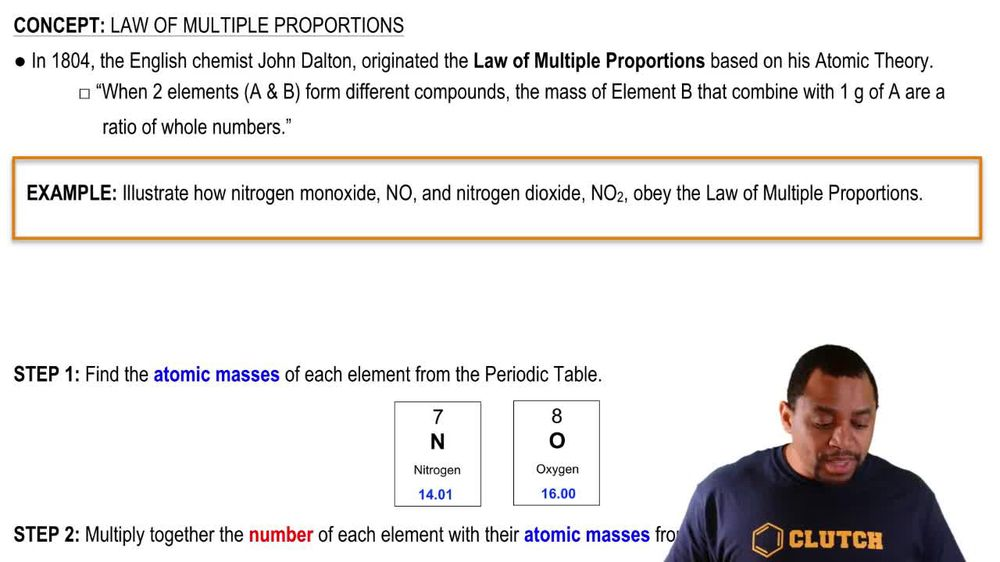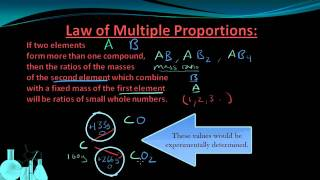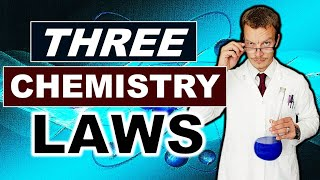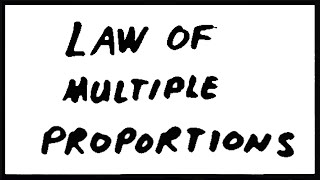Start typing, then use the up and down arrows to select an option from the list.
1. 2. Atoms & Elements2. Law of Multiple Proportions
Problem

# Ammonia (NH3) and hydrazine (N2H4) are both compounds of nitrogen and hydrogen. Based on the law of multiple pro-portions, how many grams of hydrogen would you expect 2.34 g of nitrogen to combine with to yield ammonia? To yield hydrazine?

Relevant Solution2m
Play a video:
Hello everyone today. We are being given the falling problem. Methane and ethylene are only composed of carbon and hydrogen. How much hydrogen would react with 1.78 g of carbon to produce methane and to produce ethylene. Use the law of multiple proportions. So this is a multi step problem first. We're going to solve For the g of hydrogen from methane and that's CH four. So we're going to start with our given we have 1. g of carbon. We're going to go ahead and multiply by our mass of total hydrogen in the numerator over The mass of our one carbon here so that our units for grams of carbon can cancel out. And when we simplify this, we're going to get 0.5975 g of hydrogen. We're going to repeat that process here for ethylene, which is C two H two. We're going to take our 1.78 g of carbon. We're going to multiply by our two hydrogen is that we have here. So we have two times the mass of hydrogen in the numerator over The mass of our two carbon atoms and the denominator. And so when this settles, we're going to have . grams of hydrogen. And so these are the amounts of hydrogen needed in conjunction with our methane and ethylene respectively to create that mass. And with that we've answered the question overall. I hope this helped. And until next time